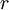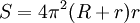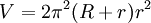# Torus

A torus is the mathematical term for a tire-like shape created by rotating a circle around the x-axis.

If the “inner radius” (the width of the doughnut hole) is, and the radius of the circular cross-section is, then, the surface area of the torus isand the volume is## Topology

In topology, a torus can be defined as the cartesian product of 2 circles. It can be constructed from a rectangle by identifying its opposite edges under the quotient topology.

This means that the torus surface is connected in the same way as the points on a rectangle, where you can “wrap-around” from one side of the rectangle to the other. This was a common topology used in the playing field of older video games.

The genus of a one-fold torus is 1.

Unusually, it is possible to divide a torus into seven different colored areas such that each area borders the other six. This is not possible on a flat surface or a sphere, where the maximum number of areas that can all touch each other is four.